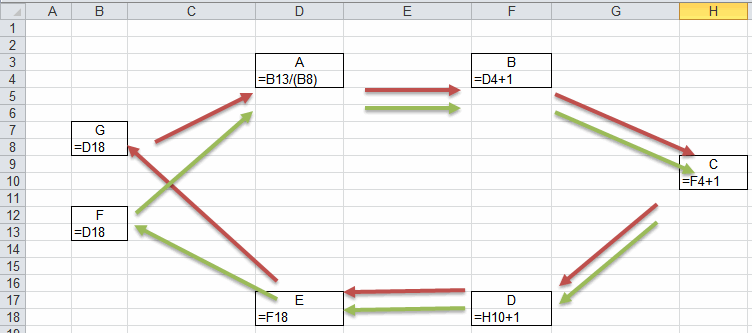1293

# Excel Circular References – How to deal with them?

## Introduction

If you’ve come to this page, chances are you’ve experienced the “Circular reference warning” popping up when you opened an Excel file or entered a formula. Excel detects a circular reference when a chain of calculations visits the same cell more than once. Many users get (very) confused by this message and have no idea what it is about. I’ll try to demystify that message here!

The next couple of pages discuss:

## Types of circular references

Simplistically speaking there are only a few types of circular references to discern:

### Deliberate circular references

The most important thing to decide up front is whether the model you are trying to calculate actually is of a circular nature. Very often there is a way to rewrite your calculations in such a way that no circular references are needed. This is always the preferred method to use.

Some people deliberately create (sets of) circular reference chains to have a calculation model do iterative calculations. Examples may include models of a chemical facility where (part of) an input stream of a process is a recycled output stream of the same process. Distillation plants often have such streams and if you want to calculate a model of such plant, using a circular reference may be a good way to model your process.

If -and only if- you are convinced you really need circular references to solve your problem, meticulously document your model, stating clearly what your intentions are and how the model functions. Especially important: Devise a way to clearly indicate the circular reference chain.

There are some sub-types of circular references to discern. I list them here under the deliberate circular references, assuming that is the only case where it could be important knowing which subtypes there are.

#### Self-referencing cells

The simplest type of a circular reference is where a cell references itself. This is sometimes used when trying to have a value in a cell which only updates under certain circumstances. An example can be the following:

Suppose you want to keep track of when an entry was made in a certain cell, without using VBA. One way could be the use of the TODAY() function, in combination with the IF function:Self-referencing cell

As soon as an entry is made in cell A1, the IF function’s test evaluates to false, making the formula in cell B1 point to it’s own value, being the date of the last time Excel recalculated.

#### Single circles

When all cells within a circle are not part of any other circle, we have a single circular reference chain. An example of such a single circle is depicted below:A single circular chain of cells

Cell C2 uses the value of cell D3, which uses the value of cell C4, which uses the value of cell B3, which in turn uses the value of the first cell, C2, completing the circle.

#### Multiple circles

To complicate things, cells may be part of more than one circular reference chain. See the example below:Multiple circles

In the example above, there are two circles. The first circle is A-B-C-D-E-F-A (green arrows). The second one is A-B-C-D-E-G-A, the red arrows. Detecting such multiple circular chains is very difficult.

Note that circular reference circles can span multiple worksheets and even multiple workbooks (try to avoid that like the plague!). If you need a circular reference, I advise you to try to keep the cells which are included in the circle as close together as you can so they can be viewed on a single screen all at once. This makes troubleshooting and validating your model easier.

### Accidental circular references

More often, people inadvertently create a circular reference, for example by having a SUM function which includes the cell the SUM function itself resides in.

Example: In cell A10, you write this function: =SUM(A1:A10)

Of course this formula is intended to sum the values in the cells above the cell with the sum function, so the argument for the SUM function should be A1:A9.